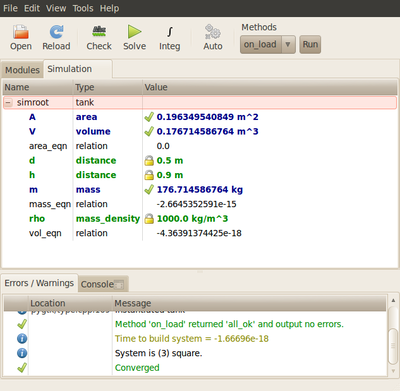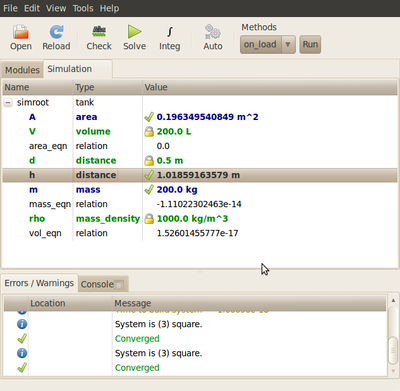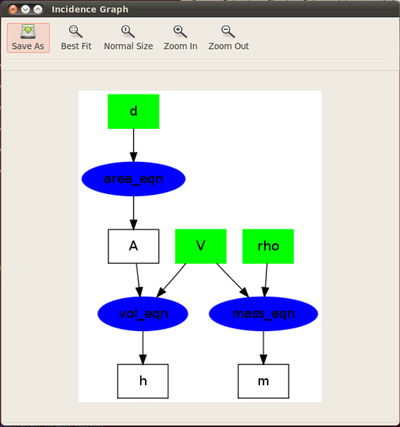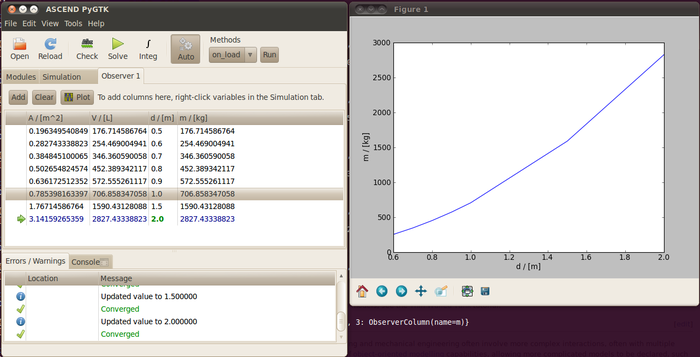# ASCEND overview

ASCEND is a system for solving systems of equations, aimed at engineers and scientists. It allows you to build up complex models as as systems constructed from simpler sub-models. Using ASCEND it is simple to play around with your model, examine its behaviour, and work out how it can best be solved. You can easily change which variables are fixed and which are to be solved, and you can examine the way in which the model is being solved.

After looking through this brief introduction to what ASCEND is all about, you might want to look at the ASCEND history, a more detailed list of ASCEND capabilities, see some worked examples, find out about the developers or look at more detailed documentation.

## Example of a simple model in ASCEND

In ASCEND, models are written in a special-purpose modelling language that combines declarative variables and equations with procedural methods. The following simple model calculates the mass of water in a tank:

```REQUIRE "atoms.a4l";

MODEL tank;
V IS_A volume;
A IS_A area;

h IS_A distance;
d IS_A distance;
area_eqn: A = 1{PI} * d^2 / 4.;

vol_eqn: V = A * h;
rho IS_A mass_density;

m IS_A mass;
mass_eqn: m = rho*V;

METHODS
FIX d, h, rho;

d := 50 {cm};
h := 90 {cm};

rho := 1000 {kg/m^3};
END tank;
```

This model declares variables and equations in the 'main' part of the model. Then, in a method called 'on_load', the model specifies that the variables d, h and rho will be 'fixed', and provides their values.

This model can be loaded into ASCEND, usually just by double-clicking the file, then solved by hitting the 'solve' button, with the following result:The simple tank model, loaded and solved in ASCEND.

This is a very simple model: there is much more that ASCEND can do. For example, without editing the above model file, one can use the GUI to 'fix' the volume and diameter of the tank and solve instead for the height, with just a couple of clicks, then resolve for a new tank volume of 200 L:You can easily 'free' and 'fix' variables to solve the same model for a different set of unknowns.

For models like this, ASCEND can quickly show you how it's solving the equation, using a simple graph representation:For small models, ASCEND can generate a graphical representation of the solution process, useful when debugging.

Simple models like this can be useful and quite efficient ways to inspect the behaviour of simple systems of equations. They can also be useful as quick 'calculators': they can be loaded, the known variables typed in, and the solved results quickly seen.

ASCEND provides a way to keep tables of 'observed' values and plot relationships between variables:The 'observer' tab allows results for a set of scenarios to be calculated and stored, and then plotted.

Note that ASCEND is dealing with units of measurement clearly and transparently. In ASCEND you never have to worry about 'getting the units right' because it understands how to convert between different units like in, ft, cm, m, km for length and L, m³ etc for volume.

To learn more about the ASCEND modelling language and how it can be used to build up models, see the Thin Walled Tank tutorial.

## More complex models

Simple models like this are useful, but serious problems, especially in process engineering and mechanical engineering often involve more complex interactions, often with multiple subsystems, interconnected parts, and so on. In this case, ASCEND provides a number of object-oriented modelling capabilities, allowing more complicated models to be declared, such as this simple steam power station (Rankine cycle) simulation:

```REQUIRE "johnpye/rankine.a4c";
MODEL power_station;

(* sub-models *)
BO IS_A boiler_simple;
TU IS_A turbine_simple;

CO IS_A condenser_simple;
PU IS_A pump_simple;

(* variable 'alias' for convenience *)

mdot ALIASES TU.mdot;

(* connections *)
BO.outlet, TU.inlet ARE_THE_SAME;

TU.outlet, CO.inlet ARE_THE_SAME;
CO.outlet, PU.inlet ARE_THE_SAME;

PU.outlet, BO.inlet ARE_THE_SAME;

(* overall cycle efficiency *)
eta IS_A fraction;

eta * (BO.Qdot_fuel - PU.Wdot) = TU.Wdot;

METHODS
METHOD specify;
RUN ClearAll;
FIX PU.outlet.p, BO.outlet.T, PU.inlet.p, PU.inlet.h, TU.eta, PU.eta, BO.eta, mdot;

END specify;
METHOD values;
mdot := 900 {t/d};

PU.outlet.p := 2000 {psi};
BO.outlet.T := 1460 {R};   BO.outlet.h := 3400 {kJ/kg};

PU.inlet.p := 1 {psi};     PU.inlet.h := 69.73 {btu/lbm};

TU.eta := 1.0;             PU.eta := 1.0;             BO.eta := 1.0;

END values;
RUN specify;
RUN values;

END power_station;
```

This model makes use of several sub-parts such as 'pump_simple' and so on, which are defined in another file called 'rankine.a4c' pointed to via the REQUIRE statement (see models/johnpye/rankine.a4c for the full code). More documentation about the syntax is available, or you can look at our other models in this area of energy system modelling.

Using this style of modular model, it is possible to build and test simple component models, then 'wire them up' into more complex models. This is something that is very difficult to do with standard spreadsheet models as commonly used by engineers. With a library of standard component models at your disposal, you can get working sooner on the complex problems which you have before you.

See Object-oriented modelling for a summary of language features that help in writing more complex models. You might also be interested in our thoughts about Modelling with Spreadsheets.

## Solvers and integrators

The basic engineering calculation being represented in the above examples is a simple "solve for 'x'" type of problem, for which ASCEND uses its QRSlv solver. For these problems ASCEND orders the equations, then uses Newton's method to iteratively solve any sets of simultaneous equations present in the system. However not all engineering problems are like this. Other types of problems can also be solved using ASCEND:

• Optimisation problems, where an objective value must be minimised by varying a set of input variables (see CONOPT, IPOPT)
• ODE and DAE problems, where the behaviour of a time-varying system is to be studied (see LSODE, IDA)
• Conditional models, where the applicable equations depend on the value or values of other variables in the model (see CMSlv)

The ASCEND GUI allows you to load a model then select a suitable 'solver' or 'integrator' according to the type of problem that you have. Several solvers are already included with ASCEND, and the API allows you to write new solvers without having to dig into the innards of ASCEND.

ASCEND can show you the model structure as 'viewed' by the solver. For example, using QRSlv, the simple Rankine-cycle model has the following lower-triangular incidence matrix structure, which indicates that no iterative solving is needed:

## Thermodynamics

An area where process engineers often run into difficulty with modelling is in incorporating the thermodynamic properties of fluids in their models. ASCEND provides some simple thermodynamics capabilities, which have proven sufficient for modelling distillation and flash problems in chemical engineering, and also integrates with high-accuracy IAPWS-IF97 steam tables for use in power engineering applications.

## Extending ASCEND

ASCEND can be extended in a number of ways, using external libraries. These are pieces of code that ASCEND loads on request. They can be:

• External Methods, doing procedural stuff to models
• external relations, adding new equations to the model, such as complex thermodynamic property relationships
• External Solvers, that solve a model, according to the programmer's definition of 'solve' :-)
• external Integrators, that integrate a model (ie solve for the dynamic response of a system; solve an inital value problem)

External libraries are normally written in C/C++, but we have implemented support for also writing external methods using Python.

## Scripting and automation

ASCEND exposes its user interface at several different levels: C, C++ and Python. Usefully, this means that if you have a more complicated modelling problem than can be solved with the ASCEND GUI, you can write a Python script that loads your model and solves it in various ways: effectively you can 'drive' ASCEND however you want, automatically.

## Development of ASCEND

ASCEND does enough things to be genuinely useful for engineers, students and scientists, and has an active user group. It was first written around 1978, and has been through several re-writes over that time. Its 'compiler' (which converts the input file into a tree structure containing variables, submodels, and equations) as well as its solvers have been the subject of several PhDs and many features in ASCEND have since been incorporated into commercial simulation programs. Development of ASCEND continues in exciting new directions however. We are working on adding new solvers as well as improving ASCEND's support for solving ODEs and DAEs.

If you are interested in contributing to ASCEND, please see our Development Activities page and also our list of Student Projects for GSOC.